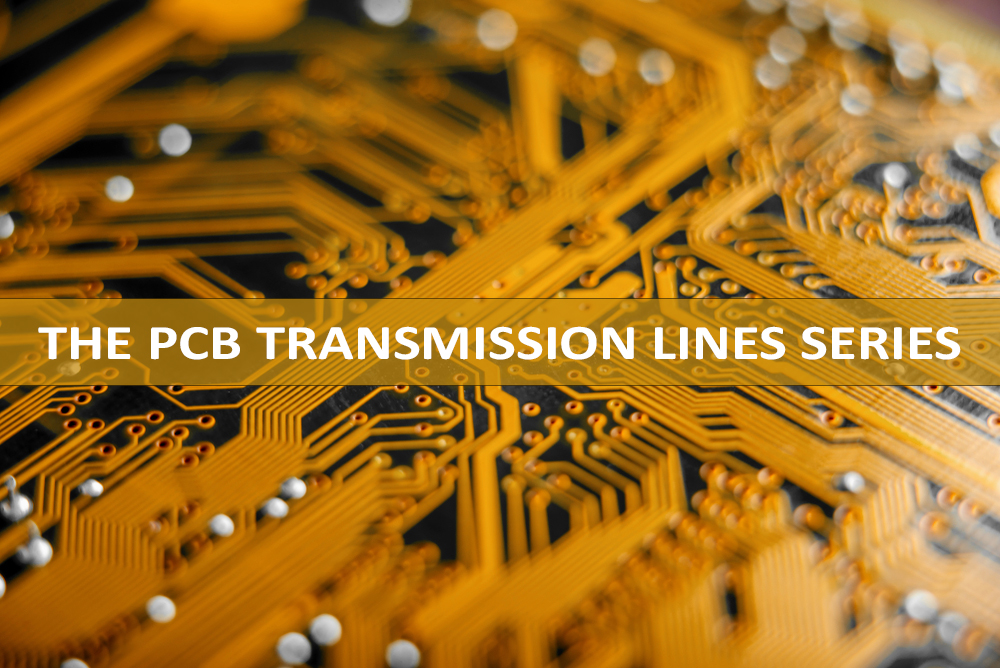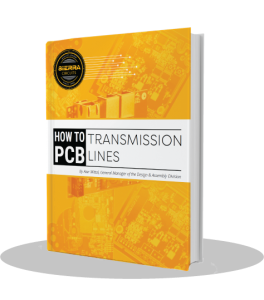# Differential Pairs in PCB Transmission Lines: Common Mode Signals## In this first part, we will discuss:

• Single-ended line
• Differential pair line
• Coupling coefficient
• Differential and common mode signals
• Differential and odd mode signals

### Single-Ended Line

In our PCB Transmission Line series, we established that a single-ended transmission line can be modeled as follows:

The relation between the voltage and current at any point on the line is given by:

Where ‘Z0’ is the characteristic (or instantaneous) impedance of the line. For a lossless or almost lossless line, we saw that ‘Z0’ is given by:

Where ‘L0’ and ‘C0’ are respectively the inductance and capacitance per unit length (pul) of the line.

#### Differential Pair Line

A pair of lines can be modeled as follows:

We will assume here that both of the lines of the pair are identical and uniform. And that they have the same separation from each other along the entire length of the line. These are precisely the characteristics of a pair of lines to be designated as a differential pair.

When we have a pair of lines close to each other, it is fair to say that the presence of a current in line 2 will induce some voltage in line 1 and a current in line 1 will induce some voltage in line 2. Thus, the voltage ‘V1’ at line 1 will not only depend on current ‘I1’ in line 1 (through impedance ‘Z0’ of line 1). It will also depend on current ‘I2’ in line 2 through coupling or mutual impedance ‘Zm’ between lines 1 and 2. This situation can be expressed by the following equation:

Where ‘Zse’ is the characteristic impedance of line 1 and ‘Zm’ is the mutual or coupling impedance between line 1 and line 2.

#### Coupling Between The Two Lines

Similarly for line 2 (of the differential pair), being identical to line 1, we can write the following equation:

The mutual impedance ‘Zm’ arises because of coupling between the two lines. And the most important coupling agents are ‘Lm’, the mutual inductance pul and ‘Cm’, the mutal capacitance pul between lines 1 and 2.

Closer are the two lines to each other, greater is the coupling between them. In fact, if the separation ‘S’ between the lines is reduced, the values of all three parameters – ‘Lm’, ‘Cm’ and ‘Zm’ – increase.

The equations (3) and (4) are true for any point on the line 1 and the corresponding point on the line 2. And for a uniform differential pair, the ‘Zse’ and ‘Zm’ have the same value at every location along the differential pair.

#### Coupling Coefficient

Since ‘Zm’ provides the magnitude of the signal voltage coupled from one line to another, as compared to the signal contributed through its own ‘Zse’, we may define the ratio ‘Zm/Zse’ as the coupling coefficient between the two lines of a differential pair:

### Differential and Common Mode Signals

#### Odd and Even Modes

Let ‘V1’ and ‘V2’ be the signal voltages and ‘I1’ and ‘I2’ be the signal currents in the two lines of a differential pair characterized by impedances ‘Zse’ and ‘Zm’. We know these six quantities are related through equations (3) and (4).

The difference in signal voltage ‘V1’ and ‘V2’ is called the differential signal ‘Vdiff’. Half of it is also called the odd mode signal:

The average value of ‘V1’ and ‘V2’ is called the common mode signal ‘Vcom’. It is also called the even mode signal:

From 5 and 6, we can express ‘V1’ and ‘V2’ in terms of ‘Vdiff’ and ‘Vcom’ as follows:

These equations indicate the universal fact that any two arbitrary signal values ‘V1’ and ‘V2’ can always be expressed as and therefore analyzed in terms of a common (or even) mode signal and a differential (or odd) mode signal.

Furthermore, the equations (7a) and (7b) also allow us to think that ‘Vcom’ or ‘Veven’ part of the signals in ‘V1’ and ‘V2’ are a kind of ‘’bias’’ on top of which the differential mode (or odd mode) signals ‘+Vodd’ and ‘-Vodd’ ride to result in ‘V1’ and ‘V2’. This viewpoint is the most important aspect of differential signaling analysis.

#### Propagating Time-Varying Signals

At this point, before we proceed further in our conceptual analysis, let’s keep in mind that the use of transmission lines – single-ended or differential – is to propagate time varying signals – usually high-speed digital signals or high-frequency analog signals – from one place to another. It is time varying signals that constitute information. Static voltages and currents do not have any information.

Therefore, looking at equations (7a) and (7b) above, we need to emphasize that ‘Vcom’ (or ‘Veven’) is only a de bias voltage on the two lines of a differential pair. The main signal is the differential signal (‘V1 – V2’). Half of which is added to line 1, usually called the positive line. It is identified by the suffix ‘+’ or ‘P’ in the name of the signal on it. The other half is subtracted from line 2, usually called the negative line and identified by the suffix ‘-‘ or ‘N’ in the name of the signal on it, to constitute ‘V1’ and ‘V2’ at the signal transmitter end.

#### At The Destination

At the destination, the two lines go to the inputs of a differential receiver which detects the difference (‘V1 – V2’) in the amplitude of the signals on the two lines as the true signal. Thereby, in the process rejecting any common mode signal – either deliberate AC bias and/or common mode noise.

This ability of rejecting the common mode signal and thus any common mode noise in differential signaling makes it far superior to single-ended signaling, where there is no way to separate the noise from the actual signal.

Having stated this, we may be tempted to think that we need to analyze deeper only the differential or odd mode and ignore the common mode. But let’s not forget that as signals propagate on the lines, they are suspect to all kinds of noise that gets superimposed on them. This may affect signal integrity adversely. Therefore, while differential pair lines’ response to the differential (ie. odd) part of the signal is our main concern, we must also analyze the differential pair’s response to the common (or even) mode signal.

#### Differential and Odd Mode Signals

We will now analyze the differential pair when we have sent only odd mode signals on it – without any common mode part.

In this case, since ‘Vcom = Veven = 0’, we have from (7a) and (7b):

Since the lines are identical, we will have ‘I2 = -I’ so that ‘I1 + I2 = 0’. Thus, there will be zero current in the return path.

Equation (3) or (4) now gives us:

We, as usual, define the ratio of ‘Vodd/Iodd’ as the odd mode impedance of the line:

Now ‘Zm = K.Zse’ where ‘K’ is the coefficient of coupling.

From this, it is clear that odd mode impedance is less than the single-ended impedance ‘Zse’ of the single line and greater the ‘Zm’ (or coupling between the two lines of the pair) is, lesser will ‘Zodd’ be from ‘Z0’.

In our next article, we will discuss differential impedance and even or common mode.Tags:

# Differential Pairs in PCB Transmission Lines: Common Mode Signals

1.KurZer says:

Hello,

shouldn´t it be Vcom=Veven=(V1+V2)2?